MATLAB Function Referenceinterp1

One-dimensional data interpolation (table lookup)

Syntax

• ```yi = interp1(x,Y,xi)
yi = interp1(Y,xi)
yi = interp1(x,Y,xi,method)
yi = interp1(x,Y,xi,method,'extrap')
yi = interp1(x,Y,xi,method,extrapval)
pp = interp1(x,Y,method,'pp')
```

Description

`yi = interp1(x,Y,xi)` interpolates to find `yi`, the values of the underlying function `Y` at the points in the vector or array `xi`. `x` must be a vector. Y can be a scalar, a vector, or an array of any dimension, subject to the following conditions:

• If `Y` is a scalar or vector, it must have the same length as `x`. A scalar value for `x` or `Y` is expanded to have the same length as the other. `xi` can be a scalar, a vector, or a multidimensional array, and `yi` has the same size as `xi`.
• If `Y` is an array that is not a vector, the size of `Y` must have the form `[n,d1,d2,...,dk]`, where `n` is the length of `x`. The interpolation is performed for each `d1`-by-`d2`-by-...-`dk `value in `Y`. The sizes of `xi` and `yi` are related as follows:
• If `xi` is a scalar or vector, `size(yi)` equals `[length(xi), d1, d2, ..., dk]`.
• If `xi` is an array of size `[m1,m2,...,mj]`, `yi` has size `[m1,m2,...,mj,d1,d2,...,dk]`.

```yi = interp1(Y,xi) ``` assumes that `x = 1:N`, where `N` is the length of `Y` for vector `Y`, or `size(Y,1)` for matrix `Y`.

```yi = interp1(x,Y,xi,method) ``` interpolates using alternative methods:

 `'nearest'` Nearest neighbor interpolation `'linear'` Linear interpolation (default) `'spline'` Cubic spline interpolation `'pchip'` Piecewise cubic Hermite interpolation `'cubic'` (Same as '`pchip'`) `'v5cubic'` Cubic interpolation used in MATLAB 5

For the `'nearest'`, `'linear'`, and `'v5cubic'` methods, `interp1(x,Y,xi,method)` returns `NaN` for any element of `xi` that is outside the interval spanned by `x`. For all other methods, `interp1` performs extrapolation for out of range values.

```yi = interp1(x,Y,xi,method,'extrap') ``` uses the specified method to perform extrapolation for out of range values.

```yi = interp1(x,Y,xi,method,extrapval) ``` returns the scalar `extrapval` for out of range values. `NaN` and `0` are often used for `extrapval`.

`pp = interp1(x,Y,method,'pp')` uses the specified method to generate the piecewise polynomial form (ppform) of `Y`. You can use any of the methods in the preceding table, except for `'v5cubic'`.

The `interp1` command interpolates between data points. It finds values at intermediate points, of a one-dimensional function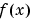that underlies the data. This function is shown below, along with the relationship between vectors `x`, `Y`, `xi`, and `yi`.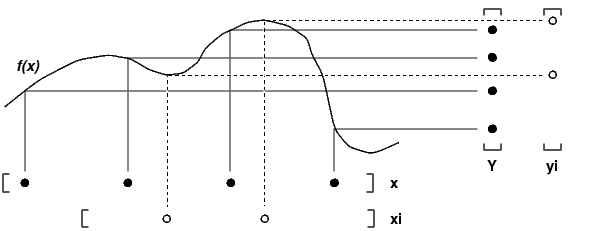Interpolation is the same operation as table lookup. Described in table lookup terms, the table is `[x,Y]` and `interp1` looks up the elements of `xi` in `x`, and, based upon their locations, returns values `yi` interpolated within the elements of `Y`.

 Note    `interp1q` is quicker than `interp1` on non-uniformly spaced data because it does no input checking. For `interp1q` to work properly, `x` must be a monotonically increasing column vector and `Y` must be a column vector or matrix with `length(X)` rows. Type `help interp1q` at the command line for more information.

Examples

Example 1. Generate a coarse sine curve and interpolate over a finer abscissa.

• ```x = 0:10;
y = sin(x);
xi = 0:.25:10;
yi = interp1(x,y,xi);
plot(x,y,'o',xi,yi)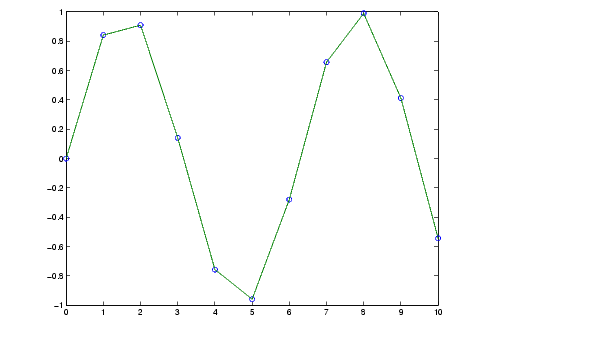```

Example 2. The following multidimensional example creates 2-by-2 matrices of interpolated function values, one matrix for each of the three functions x2, x3, and x4.

• ```x = [1:10]'; y = [ x.^2, x.^3, x.^4 ];
xi = [1.5, 1.75; 7.5, 7.75];
yi = interp1(x,y,xi);
```

The result `yi` has size 2-by-2-by-3.

• ```size(yi)

ans =

2     2     3
```

Example 3. Here are two vectors representing the census years from 1900 to 1990 and the corresponding United States population in millions of people.

• ```t = 1900:10:1990;
p = [75.995  91.972  105.711  123.203  131.669...
150.697  179.323  203.212  226.505  249.633];
```

The expression `interp1(t,p,1975)` interpolates within the census data to estimate the population in 1975. The result is

• ```ans =
214.8585
```

Now interpolate within the data at every year from 1900 to 2000, and plot the result.

• ``` x = 1900:1:2000;
y = interp1(t,p,x,'spline');
plot(t,p,'o',x,y)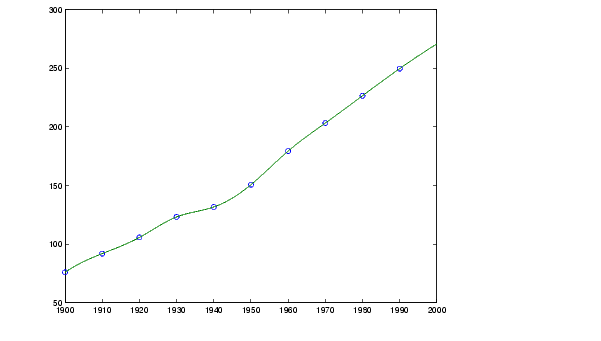```

Sometimes it is more convenient to think of interpolation in table lookup terms, where the data are stored in a single table. If a portion of the census data is stored in a single 5-by-2 table,

• ```tab =
1950    150.697
1960    179.323
1970    203.212
1980    226.505
1990    249.633
```

then the population in 1975, obtained by table lookup within the matrix `tab`, is

• ```p = interp1(tab(:,1),tab(:,2),1975)
p =
214.8585
```

Example 4. The following example uses the `'cubic'` method to generate the piecewise polynomial form (ppform) of `Y`, and then evaluates the result using `ppval`.

• ```x = 0:.2:pi; y = sin(x);
pp = interp1(x,y,'cubic','pp');
xi = 0:.1:pi;
yi = ppval(pp,xi);
plot(x,y,'ko'), hold on, plot(xi,yi,'r:'), hold off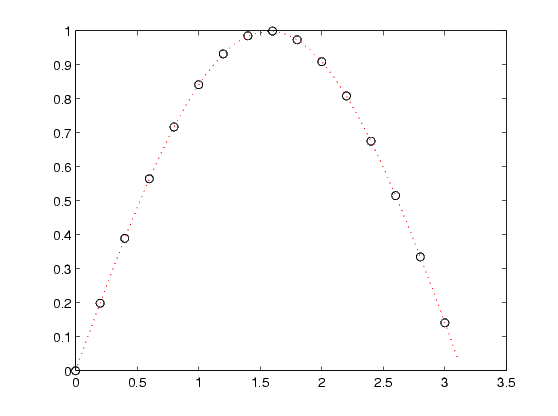```

Algorithm

The `interp1` command is a MATLAB M-file. The '`nearest'` and '`linear'` methods have straightforward implementations.

For the '`spline'` method, `interp1` calls a function `spline` that uses the functions `ppval`, `mkpp`, and `unmkpp`. These routines form a small suite of functions for working with piecewise polynomials. `spline` uses them to perform the cubic spline interpolation. For access to more advanced features, see the `spline` reference page, the M-file help for these functions, and the Spline Toolbox.

For the '`pchip'` and `'cubic'` methods, `interp1` calls a function `pchip` that performs piecewise cubic interpolation within the vectors `x` and `y`. This method preserves monotonicity and the shape of the data. See the `pchip` reference page for more information.

See Also

`interpft`, `interp2`, `interp3`, `interpn`, `pchip`, `spline`

References

  de Boor, C., A Practical Guide to Splines, Springer-Verlag, 1978.int8, int16, int32, int64 interp2© 1994-2005 The MathWorks, Inc.